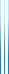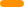# PublicationsPapers

## Low-degree Boolean functions on $S_n$, with an application to isoperimetry

David Ellis, Yuval Filmus and Ehud Friedgut
Forum of Mathematics, Sigma, Volume 5, 2017

We prove that Boolean functions on $S_n$ whose Fourier transform is highly concentrated on irreducible representations indexed by partitions of $n$ whose largest part has size at least $n-t$ are close to being unions of cosets of stabilizers of $t$-tuples. We also obtain an edge-isoperimetric inequality for the transposition graph on $S_n$ which is asymptotically sharp for sets of measure $1/\mathit{poly}(n)$. We then combine both results to obtain a best-possible edge-isoperimetric inequality for sets of size $(n-t)!$ where $n$ is large compared to $t$, confirming a conjecture of Ben-Efraim in these cases.

## BibTeX

@article{EFF3,
author = {David Ellis and Yuval Filmus and Ehud Friedgut},
title = {Low-degree {B}oolean functions on {$S_n$}, with an application to isoperimetry},
journal = {Forum of Mathematics, Sigma},
volume = {5},
publisher = {Cambridge University Press},
DOI = {10.1017/fms.2017.24},
year = {2017}
}copy to clipboard